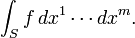# Integrals of differential forms

A differential form is a mathematical concept in the fields of multivariable calculus, differential topology and tensors. The modern notation for the differential form, as well as the idea of the differential forms as being the wedge products of exterior derivatives forming an exterior algebra, was introduced by Élie Cartan.

We initially work in an open set in Rn. A 0-form is defined to be a smooth function f. When we integrate a function f over an m-dimensional subspace S of Rn, we write it as(The superscripts are indices, not exponents.) We can consider dx1 through dxn to be formal objects themselves, rather than tags appended to make integrals look like Riemann sums. Alternatively, we can view them as covectors, and thus a measure of “density” (hence integrable in a general sense). We call the dx1, …,dxn basic 1-forms.

We define the wedge product, “∧”, a bilinear “multiplication” operator on these elements, with the alternating property thatfor all indices a. Note that alternation along with linearity and associativity implies dxbdxa = −dxadxb. This also ensures that the result of the wedge product has an orientation.

We define the set of all these products to be basic 2-forms, and similarly we define the set of products of the form dxadxbdxc to be basic 3-forms. A general k-form is then a weighted sum of basic k-forms, where the weights are the smooth functions f. Together these form a vector space with basic k-forms as the basis vectors, and 0-forms (smooth functions) as the field of scalars. The wedge product then extends to k-forms in the natural way. Over Rn at most n covectors can be linearly independent, thus a k-form with k > n will always be zero, by the alternating property.

In addition to the wedge product, there is also the exterior derivative operator d. This operator maps k-forms to (k+1)-forms. For a k-form ω = f dxa over Rn, we define the action of d by:with extension to general k-forms occurring linearly.

This more general approach allows for a more natural coordinate-free approach to integration on manifolds. It also allows for a natural generalisation of the fundamental theorem of calculus, called Stokes’ theorem, which we may state aswhere ω is a general k-form, and ∂Ω denotes the boundary of the region Ω. Thus, in the case that ω is a 0-form and Ω is a closed interval of the real line, this reduces to the fundamental theorem of calculus. In the case that ω is a 1-form and Ω is a two-dimensional region in the plane, the theorem reduces to Green’s theorem. Similarly, using 2-forms, and 3-forms and Hodge duality, we can arrive at Stokes’ theorem and the divergence theorem. In this way we can see that differential forms provide a powerful unifying view of integration.

RELATED POST ::Welcome to my blog. My name is Nico. Admin of this blog. I am a student majoring in mathematics who dreams of becoming a professor of mathematics. I live in Kwadungan, Ngawi, East Java. Hopefully in all the posts I can make a good learning material to the intellectual life of the nation. After the read, leave a comment. I always accept criticism suggestion to build a better me again .. Thanks for visiting .. : mrgreen:

Posted on September 5, 2011, in education and tagged , , , , , , , . Bookmark the permalink. 2 Comments.

1.Marchei Riendra
•NICO VENGEANCE